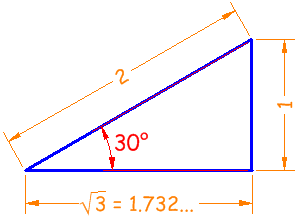# Sohcahtoa

Sohca...what? Just an easy way to remember how Sine, Cosine and Tangent work:

 Soh... Sine = Opposite / Hypotenuse ...cah... Cosine = Adjacent / Hypotenuse ...toa Tangent = Opposite / Adjacent

## Right Triangle

OK, let's see what this is all about.

Firstly, the names Opposite, Adjacent and Hypotenuse come from the right triangle:• "Opposite" is opposite to the angle θ
• "Hypotenuse" is the long oneAdjacent is always next to the angle

And Opposite is opposite the angle

## Sine, Cosine and Tangent

And Sine, Cosine and Tangent are the three main functions in trigonometry.

They are often shortened to sin, cos and tan.

The calculation is simply one side of a right angled triangle divided by another side ... we just have to know which sides, and that is where "sohcahtoa" helps.

For a triangle with an angle θ , the functions are calculated this way:

 Sine: soh... sin(θ) = opposite / hypotenuse Cosine: ...cah... cos(θ) = adjacent / hypotenuse Tangent: ...toa tan(θ) = opposite / adjacent

### Example: what are the sine, cosine and tangent of 30° ?

A 30° triangle has a hypotenuse (the long side) of length 2, an opposite side of length 1 and an adjacent side of √3, like this:Now we know the lengths, we can calculate the functions:

 Sine soh... sin(30°) = 12 = 0.5 Cosine ...cah... cos(30°) = 1.732...2 = 0.866... Tangent ...toa tan(30°) = 11.732... = 0.577...

(get your calculator out and check them!)

## How to Remember

I find "sohcahtoa" easy to remember ... but here are other ways if you like:

• Sailors Often Have Curly Auburn Hair Till Old Age.
• Some Old Horses Can Always Hear Their Owners Approach.
• Some Old Hen Caught Another Hen Taking One Away.